#### Conic Sections Homework Help - K-12 Grade Level, College Level Geometry Mathematics

Introduction of Conic section

A Conic Section (or just conic) is a curve obtained as the intersection of a cone. More specifically, a right circular conical surface with a plane. In analytic geometry, a conic may be described as a plane algebraic curve of degree 2. There are a various other geometric definitions possible. One of the most useful is that a conic consists of those points whose distances to some point called a focus and some line called a directrix are in a fixed ratio called the eccentricity.

Traditionally, the three types of conic section are the hyperbola, parabola and ellipse. The circle is a special case of the ellipse and is of sufficient interest in its own right that it is sometimes called the 4th type of conic section. Type of a conic corresponds to its eccentricity, those with eccentricity less than 1 is being ellipses, those with eccentricity equal to 1 is being parabolas and those with eccentricity greater than 1 is being hyperbola. In the focus-directrix definition of a conic the circle is a limiting case with eccentricity 0. In modern geometry there are degenerate cases like the union of two lines is included as conics as well.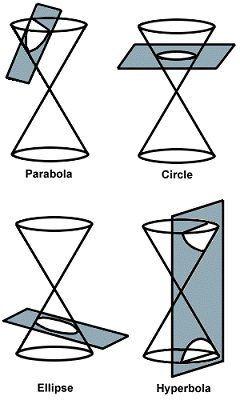conic section equation eccentricity (e) linear eccentricity (c) semi-latus rectum (l) focal parameter (p) circle X2+y2=a2 0 0 a ∞ ellipse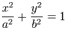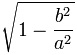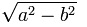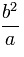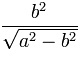parabola Y2=4ax 1 a 2a 2a hyperbola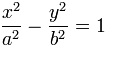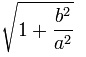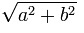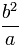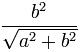Conics – General Information

What is Conics, or conic sections, are explained as plane figures that are shaped while it is intersected with a double-napped cone and a plane.  The below diagram shows the unlike conics that may be formed by a double-napped cone being cut by a plane: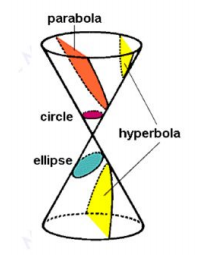Parabolas:  It is noticed that to create a parabola through intersecting a cone and a plane, the plane can pass through the base of the cone, but only will pass through one of the cones.

Any of these conics may be graphed on a coordinate plane.  Thus the graph of any conic on an x-y coordinate plane can be mentioned by an equation in the form:

Ax2 + Bxy + Cy2 + Dx + Ey + F = 0

This is called equation of General Form for all conics.  We study the different conics and their equations, graphs, that we may conclude the type of conic mentioned the equation based on the values of the given coefficients, A, B, C, D, E and F.

Email based Conic Section Homework Help -Assignment Help

Tutors at the www.tutorsglobe.com are committed to provide the best quality Conic Section homework help - assignment help. They use their experience, as they have solved thousands of the Conic Section assignments, which may help you to solve your complex Conic Section homework. You can find solutions for all the topics come under the Conic Section. The dedicated tutors provide eminence work on your Geometry homework help and devoted to provide K-12 level Geometry Mathematics to college level Geometry Mathematics help before the deadline mentioned by the student. Conic Section homework help is available here for the students of school, college and university. Tutors Globe assure for the best quality compliance to your homework. Compromise with quality is not in our dictionary. If we feel that we are not able to provide the homework help as per the deadline or given instruction by the student, we refund the money of the student without any delay.

Qualified and Experienced Conic Section Tutors at www.tutorsglobe.com

Tutors at the www.tutorsglobe.com take pledge to provide full satisfaction and assurance in Conic Section homework help. Students are getting Geometry Mathematics homework help services across the globe with 100% satisfaction. We value all our service-users. We provide email based Conic Section homework help - assignment help. You can join us to ask queries 24x7 with live, experienced and qualified Geometry Mathematics tutors specialized in Conic Section.